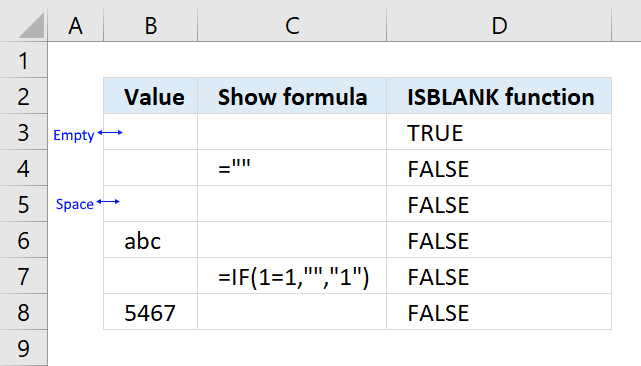Author: Oscar Cronquist Article last updated on April 17, 2018The ISBLANK function returns TRUE if the argument is an empty cell, returns FALSE if not.

ISBLANK(value)

### Arguments

 value Required. The cell you want to check if empty.

### Comments

The ISBLANK function returns FALSE if a formula returns nothing, see picture above in cell B4 and B7.

To check if a formula returns nothing use the following formula:

=IF(B3="",TRUE,FALSE)

This returns TRUE if a formula returns nothing "".